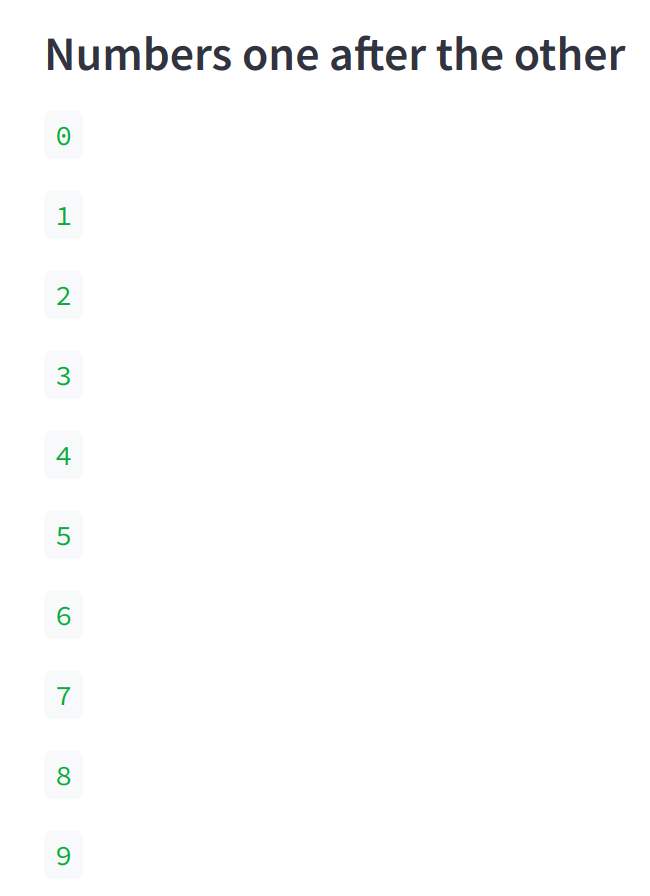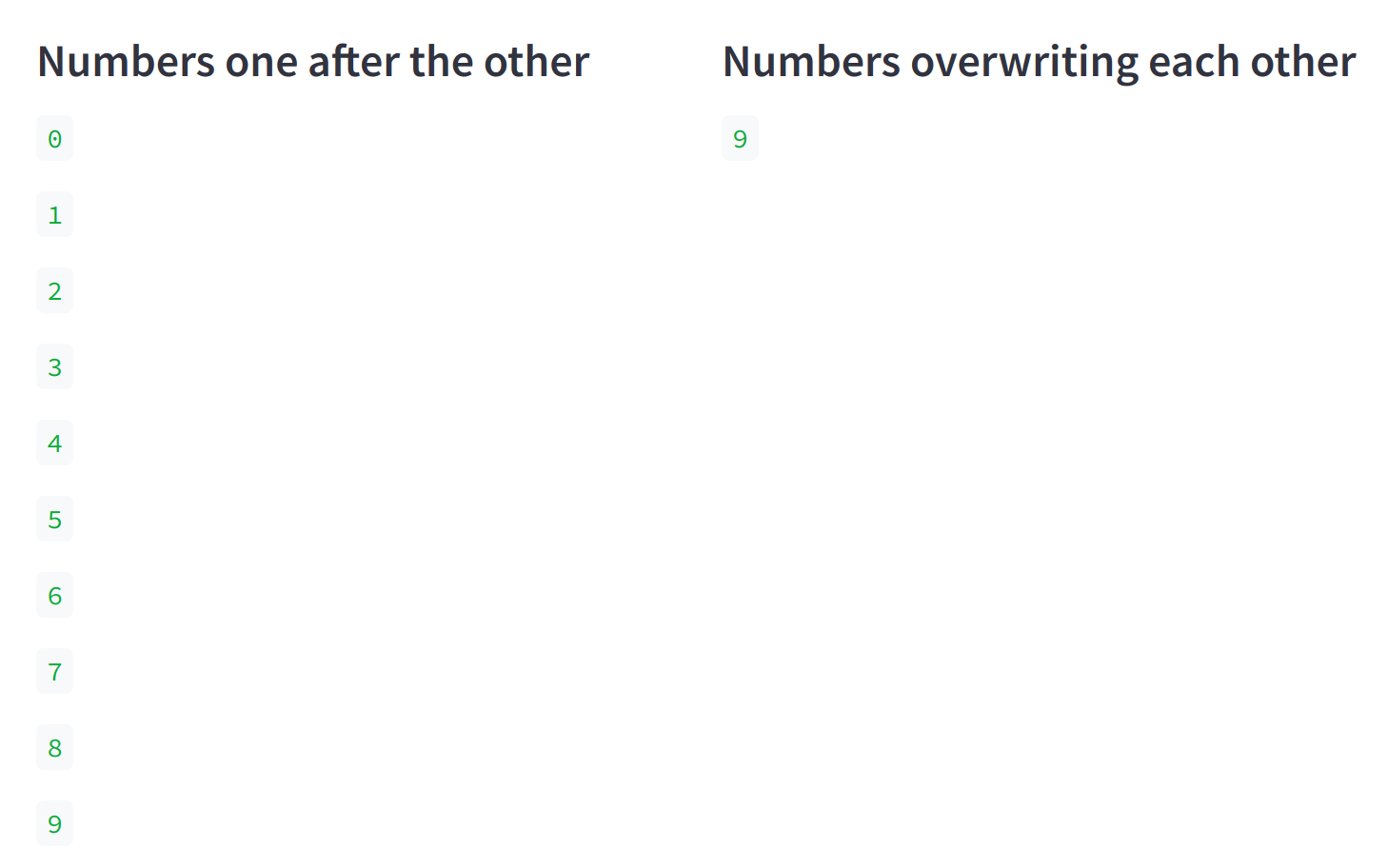# Streamlit: Overwrite previous value in a loop

I was recently building a Streamlit app in which I was looping through a stream of values and wanted to only print out the most recent value. In this blog post we’ll learn how to do that.Figure 1. Streamlit: Overwrite previous value in a loop

## Setup

If you want to play along you’ll need to create a virtual environment and install Streamlit:

``````python -m  venv env
source venv/bin/activate
pip install streamlit``````

## Streamlit App

Now, create a file `app.py` that contains the following:

app.py
``````import streamlit as st

st.markdown("#### Numbers one after the other")
for i in range(0,10):
st.write(i)``````

And run the following command from the terminal:

``streamlit run app.py``

If we run this command it will open a web browser at http://localhost:8501, and we’ll see the following output:Figure 2. Numbers one after the other

The numbers are all printed out, which isn’t exactly what we want. In this example we’re only printing out 10 numbers, but if we had a bigger stream of values it would eventually crash the browser!

Instead we can use a single element container (`st.empty`) and populate that on each loop. Let’s update `app.py` to look like this:

app.py
``````import streamlit as st

left, right = st.columns(2)

with left:
st.markdown("#### Numbers one after the other")
for i in range(0,10):
st.write(i)

with right:
st.markdown("#### Numbers overwriting each other")
numbers = st.empty()

for i in range(0,10):
with numbers.container():
st.write(i)``````

Now if we go back to our Streamlit app, we’ll see the following:Figure 3. Numbers overwriting each other

We can only see the number 9 because that’s the last value that got printed.

If we want to see how the output progresses, we’ll need to pause the loop for a little bit after each iteration. Update `app.py` to look like this:

app.py
``````import streamlit as st
import time

left, right = st.columns(2)

with left:
st.markdown("#### Numbers one after the other")
for i in range(0,10):
st.write(i)

with right:
st.markdown("#### Numbers overwriting each other")
numbers = st.empty()

for i in range(0,10):
with numbers.container():
st.write(i)
time.sleep(0.5)``````

And now if we refresh the page, we’ll see it update like this:Figure 4. Numbers overwriting each other animation

Exactly what I wanted - good work Streamlit!